# How do I Solve this Equation? Look at the Symmetries! – The Idea behind Galois Theory

Originating author is Timo Leuders.

Introduction
There are some questions that accompany the development of mathematics through cultures and ages. One of these questions is how to find an unknown quantity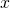of which one knows some relations such as – in today’s algebraic notation: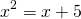Finding solutions to such quadratic equations are essentially known since Babylonian
times and are core content school mathematics: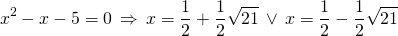But how about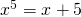, which looks only slightly different? Are there also straightforward ways to calculate the solutions? Do the solutions also look symmetric in a similar way?

The quest for solving equations inspired mathematicians to invent (some would rather say: to discover) new concepts such as negative, real or complex numbers. But, solving the polynomial equation in the second example posed severe problems for five hundred years. Why is it so difficult? Let us cheat for a moment and ask a Computer Algebra System (CAS) – which of course uses what is known about solving equations.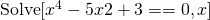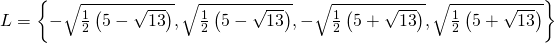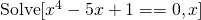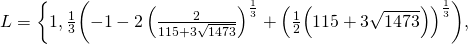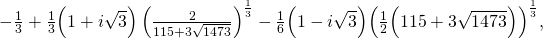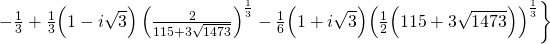Finally: For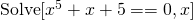the CAS gives up and yields no solution.

What is going on here? Why does a seemingly small change in the equation lead to such tremendous problems in presenting the solutions? What is the structure of the equation that ultimately decides on the existence or the complexity of a solution? The answer to these questions is: It is all about the symmetry of the equation! But what exactly is the symmetry of an equation?
The attempts in history to find a general solution procedure for polynomial equations finally lead to a transformation of classical algebra (as the art of solving equations) into modern algebra (as the analysis of structure and symmetry). A culmination point in this development was the work of Évariste Galois (1811-1832). This paper tries to give a less technical account of Galois’ ideas that changed algebra by showing examples that highlight what it means to „look at structure and symmetry“ when trying to solve equations.

[/latexpage]

This post is also available in: German, Arabic

This entry was posted in Mathematics Within the Last 100 Years. Bookmark the permalink.
1.Anonymous says:

The link to the full vignette text is broken.
Thanks